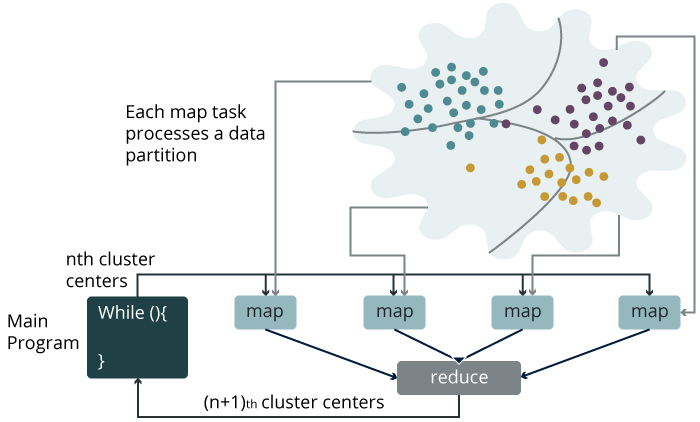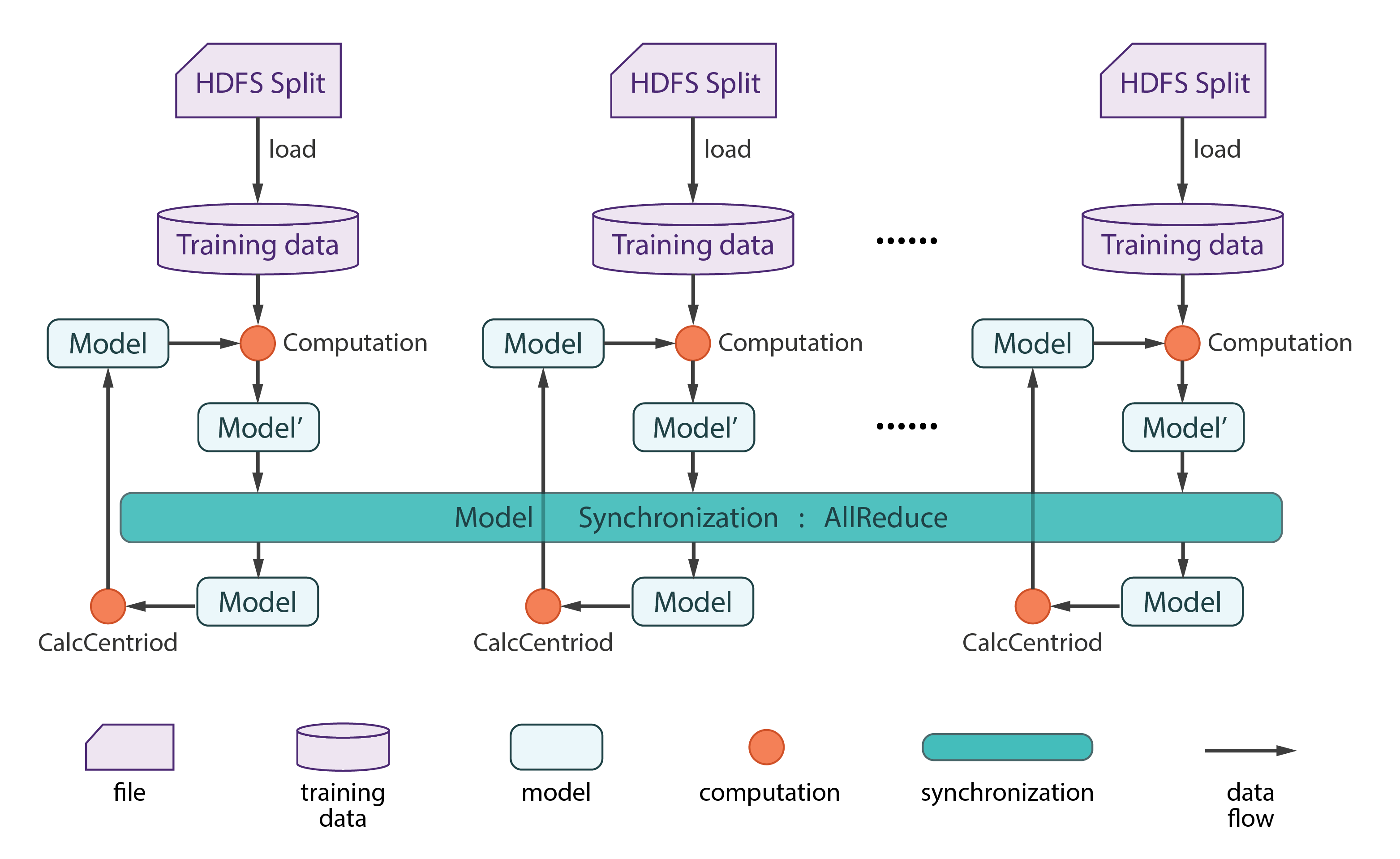Before going through this tutorial take a look at the overview section to get an understanding of the structure of the tutorial.K-Means is a powerful and easily understood clustering algorithm. The aim of the algorithm is to divide a given set of points into `K` partitions. `K` needs to be specified by the user. In order to understand K-Means, first, you need to understand the proceeding concepts and their meanings.

• `Centroids`: Centroids can be defined as the center of each cluster. If we are performing clustering with k=3, we will have 3 centroids. To perform K-Means clustering, the users need to provide an initial set of centroids.
• `Distance`: In order to group data points as close together or as far-apart, we need to define a distance between two given data points. In K-Means, clustering distance is normally calculated as the Euclidean Distance between two data points.

The K-Means algorithm simply repeats the following set of steps until there is no change in the partition assignments. In that, it has clarified which data point is assigned to which partition.

1. Choose K points as the initial set of centroids.

2. Assign each data point in the dataset to the closest centroid (this is done by calculating the distance between the data point and each centroid).

3. Calculate the new centroids based on the clusters that were generated in step 2. Normally this is done by calculating the mean of each cluster.

4. Repeat step 2 and 3 until data points do not change cluster assignments, which means that their centroids are set.

## PARALLEL DESIGN

• What are the models? What kind of data structure is applicable?

Centroids of the clusters are models in vanilla k-means. It has a vector structure as a double array.

• What are the characteristics of the data dependency in model update computation, can updates run concurrently?

In the core model update computation, each data point should access all the model, compute distance with each centroid, find the nearest one and partially update the model. The true update only occurs when all data points finish their search.

• Which kind of parallelism scheme is suitable, data parallelism or model parallelism?

Data parallelism can be used, i.e., calculating different data points in parallel.

For model parallelism, there are two different solutions.

1). Without model parallelism, each node get one replica of the whole model, which updates locally in parallel, and then synchronizes when local computation all finish

2). With model parallelism, each node gets one partition of the model, which updates in parallel, and then rotates to the neighbor node when local computation for all local data points finish. Repeat until each partition returns back to the original node, then do the final model update.

• Which collective communication operation is suitable to synchronize the model?

For solution without model parallelism, Synchronize replicas of the model by `allreduce`, then calculate the new centroids and go to the next iteration. For vanilla kmeans, the combination of `reduce/broadcast`, `push/pull`, `regroup/allgather` are similar to `allreduce`.

For solution with model parallelism, there is no replica exists, but the movement of the model partitions are a kind of synchronized collective operation, supported in Harp by an abstraction of rotate.

## DATAFLOW## Step 1 — The Main Method

The tasks of the main class are to configure and run the job iteratively.

```generate N data points (D dimensions), write to HDFS
generate M centroids, write to HDFS
for iterations{
configure a job
launch the job
}
```

## Step 2 — The mapCollective function

This is the definition of the map-collective task. It reads data from context and then calls `runKmeans` function to actually run k-means Mapper task.

```protected void mapCollective( KeyValReader reader, Context context) throws IOException, InterruptedException {
LOG.info("Start collective mapper.");
long startTime = System.currentTimeMillis();
List<String> pointFiles = new ArrayList<String>();
while (reader.nextKeyValue()) {
String key = reader.getCurrentKey();
String value = reader.getCurrentValue();
LOG.info("Key: " + key + ", Value: " + value);
pointFiles.add(value);
}
Configuration conf = context.getConfiguration();
runKmeans(pointFiles, conf, context);
LOG.info("Total iterations in master view: " + (System.currentTimeMillis() - startTime));
}
```

## Step 3 — The runKmeans function

Harp provides several collective communication operations. Here are some examples provided to show how to apply these collective communication methods to K-Means.

#### Use AllReduce collective communication to do synchronization

```private void runKmeans(List<String> fileNames, Configuration conf, Context context) throws IOException {
// —————————————————–
// Load centroids
//for every partition in the centoid table, we will use the last element to store the number of points
// which are clustered to the particular partitionID
Table<DoubleArray> cenTable = new Table<>(0, new DoubleArrPlus());
if (this.isMaster()) {
loadCentroids(cenTable, vectorSize, conf.get(KMeansConstants.CFILE), conf);
}
System.out.println("After loading centroids");
printTable(cenTable);
//broadcast centroids
broadcastCentroids(cenTable);
//after broadcasting
System.out.println("After brodcasting centroids");
printTable(cenTable);
//load data
ArrayList<DoubleArray> dataPoints = loadData(fileNames, vectorSize, conf);
Table<DoubleArray> previousCenTable =  null;
//iterations
for(int iter=0; iter < iteration; iter++){
previousCenTable =  cenTable;
cenTable = new Table<>(0, new DoubleArrPlus());
System.out.println("Iteraton No."+iter);
//compute new partial centroid table using previousCenTable and data points
computation(cenTable, previousCenTable, dataPoints);
//AllReduce;
/*************************************/
allreduce("main", "allreduce_"+iter, cenTable);
//we can calculate new centroids
calculateCentroids(cenTable);
/*************************************/
printTable(cenTable);
}
//output results
if(this.isMaster()){
outputCentroids(cenTable,  conf,   context);
}
}
```

#### Use broadcast and reduce collective communication to do synchronization

The video below is the step by step guide on how this collective communication works for K-means. The data is partitions into K different partitions with K centroids. Data is then broadcasted to all the different partitions. And the centroids for each of the partition is grouped together and sent to the master node.

Once all the local centroids from the partition is collected in the global centroid table, the updated table is transferred to the root node and then broadcasted again. This step keeps repeating itself till the convergence is reached.

```private void runKmeans(List<String> fileNames, Configuration conf, Context context) throws IOException {
// —————————————————–
// Load centroids
//for every partition in the centoid table, we will use the last element to store the number of points
// which are clustered to the particular partitionID
Table<DoubleArray> cenTable = new Table<>(0, new DoubleArrPlus());
if (this.isMaster()) {
loadCentroids(cenTable, vectorSize, conf.get(KMeansConstants.CFILE), conf);
}
System.out.println("After loading centroids");
printTable(cenTable);
//broadcast centroids
broadcastCentroids(cenTable);
//after broadcasting
System.out.println("After brodcasting centroids");
printTable(cenTable);
//load data
ArrayList<DoubleArray> dataPoints = loadData(fileNames, vectorSize, conf);
Table<DoubleArray> previousCenTable =  null;
//iterations
for(int iter=0; iter < iteration; iter++){
previousCenTable =  cenTable;
cenTable = new Table<>(0, new DoubleArrPlus());
System.out.println("Iteraton No."+iter);
//compute new partial centroid table using previousCenTable and data points
computation(cenTable, previousCenTable, dataPoints);
/*************************************/
reduce("main", "reduce"+iter, cenTable, this.getMasterID());
if(this.isMaster())
calculateCentroids(cenTable);
broadcast("main", "bcast"+iter, cenTable, this.getMasterID(), false);
/**********************************/
printTable(cenTable);
}
//output results
if(this.isMaster()){
outputCentroids(cenTable,  conf,   context);
}
}
```

#### Use push and pull collective communication to do synchronization

```private void runKmeans(List<String> fileNames, Configuration conf, Context context) throws IOException {
// —————————————————–
// Load centroids
//for every partition in the centoid table, we will use the last element to store the number of points
// which are clustered to the particular partitionID
Table<DoubleArray> cenTable = new Table<>(0, new DoubleArrPlus());
if (this.isMaster()) {
loadCentroids(cenTable, vectorSize, conf.get(KMeansConstants.CFILE), conf);
}
System.out.println("After loading centroids");
printTable(cenTable);
//broadcast centroids
broadcastCentroids(cenTable);
//after broadcasting
System.out.println("After brodcasting centroids");
printTable(cenTable);
//load data
ArrayList<DoubleArray> dataPoints = loadData(fileNames, vectorSize, conf);
Table<DoubleArray> globalTable = new Table<DoubleArray>(0,  new DoubleArrPlus());
Table<DoubleArray> previousCenTable =  null;
//iterations
for(int iter=0; iter < iteration; iter++){
// clean contents in the table.
globalTable.release();
previousCenTable =  cenTable;
cenTable = new Table<>(0, new DoubleArrPlus());
System.out.println("Iteraton No."+iter);
//compute new partial centroid table using previousCenTable and data points
computation(cenTable, previousCenTable, dataPoints);
/**********************************/
push("main", "push"+iter, cenTable, globalTable , new Partitioner(this.getNumWorkers()));
//we can calculate new centroids
calculateCentroids(globalTable);
pull("main", "pull"+iter, cenTable, globalTable, true);
/**********************************/
printTable(cenTable);
}
//output results
if(this.isMaster()){
outputCentroids(cenTable,  conf,   context);
}
}
```

### Use Regroup and allgather collective communication to do synchronization

```private void runKmeans(List<String> fileNames, Configuration conf, Context context) throws IOException {
// —————————————————–
// Load centroids
//for every partition in the centoid table, we will use the last element to store the number of points
// which are clustered to the particular partitionID
Table<DoubleArray> cenTable = new Table<>(0, new DoubleArrPlus());
if (this.isMaster()) {
loadCentroids(cenTable, vectorSize, conf.get(KMeansConstants.CFILE), conf);
}
System.out.println("After loading centroids");
printTable(cenTable);
//broadcast centroids
broadcastCentroids(cenTable);
//after broadcasting
System.out.println("After brodcasting centroids");
printTable(cenTable);
//load data
ArrayList<DoubleArray> dataPoints = loadData(fileNames, vectorSize, conf);
Table<DoubleArray> previousCenTable =  null;
//iterations
for(int iter=0; iter < iteration; iter++){
previousCenTable =  cenTable;
cenTable = new Table<>(0, new DoubleArrPlus());
System.out.println("Iteraton No."+iter);
//compute new partial centroid table using previousCenTable and data points
computation(cenTable, previousCenTable, dataPoints);
/**********************************/
//regroup and allgather to synchronized centroids
regroup("main", "regroup"+iter, cenTable, null);
//we can calculate new centroids
calculateCentroids(cenTable);
allgather("main", "allgather"+iter, cenTable);
/*************************************/
printTable(cenTable);
}
//output results
if(this.isMaster()){
outputCentroids(cenTable,  conf,   context);
}
}
```

## Step 4 — Compute local centroids

```private void computation(Table<DoubleArray> cenTable, Table<DoubleArray> previousCenTable,ArrayList<DoubleArray> dataPoints){
double err=0;
for(DoubleArray aPoint: dataPoints){
//for each data point, find the nearest centroid
double minDist = -1;
double tempDist = 0;
int nearestPartitionID = -1;
for(Partition ap: previousCenTable.getPartitions()){
DoubleArray aCentroid = (DoubleArray) ap.get();
tempDist = calcEucDistSquare(aPoint, aCentroid, vectorSize);
if(minDist == -1 || tempDist < minDist){
minDist = tempDist;
nearestPartitionID = ap.id();
}
}
err+=minDist;

//for the certain data point, found the nearest centroid.
// add the data to a new cenTable.
double[] partial = new double[vectorSize+1];
for(int j=0; j < vectorSize; j++){
partial[j] = aPoint.get()[j];
}
partial[vectorSize]=1;

if(cenTable.getPartition(nearestPartitionID) == null){
Partition<DoubleArray> tmpAp = new Partition<DoubleArray>(nearestPartitionID, new DoubleArray(partial, 0, vectorSize+1));
cenTable.addPartition(tmpAp);
}else{
Partition<DoubleArray> apInCenTable = cenTable.getPartition(nearestPartitionID);
for(int i=0; i < vectorSize +1; i++){
apInCenTable.get().get()[i] += partial[i];
}
}
}
System.out.println("Errors: "+err);
}
```

## Step 5 — Calculate new centroids

```private void calculateCentroids( Table<DoubleArray> cenTable){
for( Partition<DoubleArray> partialCenTable: cenTable.getPartitions()){
double[] doubles = partialCenTable.get().get();
for(int h = 0; h < vectorSize; h++){
doubles[h] /= doubles[vectorSize];
}
doubles[vectorSize] = 0;
}
System.out.println("after calculate new centroids");
printTable(cenTable);
}
```

## COMPILE

Select the profile related to your hadoop version. For ex: hadoop-2.6.0. Supported hadoop versions are 2.6.0, 2.7.5 and 2.9.0

```cd \$HARP_ROOT_DIR
mvn clean package -Phadoop-2.6.0
```
```cd \$HARP_ROOT_DIR/contrib/target
cp contrib-0.1.0.jar \$HADOOP_HOME
cd \$HADOOP_HOME
```

## USAGE

Run Harp K-Means:

```hadoop jar contrib-0.1.0.jar edu.iu.kmeans.common.KmeansMapCollective <numOfDataPoints> <num of Centroids> <size of
vector> <number of map tasks> <number of iteration> <workDir> <localDir> <communication operation>

<numOfDataPoints>: the number of data points you want to generate randomly
<num of centriods>: the number of centroids you want to clustering the data to
<size of vector>: the number of dimension of the data
<number of map tasks>: number of map tasks
<number of iteration>: the number of iterations to run
<work dir>: the root directory for this running in HDFS
<local dir>: the harp kmeans will firstly generate files which contain data points to local directory. Set this argument to determine the local directory.
<communication operation> includes:
[allreduce]: use allreduce operation to synchronize centroids
[regroup-allgather]: use regroup and allgather operation to synchronize centroids
[broadcast-reduce]: use broadcast and reduce operation to synchronize centroids
[push-pull]: use push and pull operation to synchronize centroids
```

For example:

```hadoop jar contrib-0.1.0.jar edu.iu.kmeans.common.KmeansMapCollective 1000 10 10 2 10 /kmeans /tmp/kmeans allreduce
```

Fetch the results:

```hdfs dfs -ls /
hdfs dfs -cat /kmeans/centroids/*
```